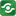ChiSquare 1@import url(http://www.google.com/cse/api/branding.css);CSGNetwork Custom SearchCSGNetwork Snippets Calculators Top Free Apps Home Back

Right click this window and select "view source" in order to copy the source for this script.

## Calculate probability from X2 and d

One of the most common chi-square calculations is determining, given the measured X2 value for a set of experiments with a degree of freedom d, the probability of the result being due to chance. Enter the X2 and d values in the boxes below, press the Calculate button, and the probability will appear in the Q box.

Given X2= and d=

The chance probability, Q, is: